IFERROR is an Excel Logical Function to Check if a value is an Error. IFERROR used in Excel to handle if the formula is evaluated to an Error. We can handle the Error Cells and Formulas with errors like #VALUE!,#N/A, #REF!, #DIV/0!, #NUM! #NAME? and #NULL!.

In this topic:

## IFERROR Function

Excel IFError Function helps to return a value if an formula returns an Error. For example, if we enter some formula in Cell, and we assume that there is some chance of getting an Error. In this case we can use IFERROR function to return something else when the formula evaluates an error.

=IFERROR(Actual Formula, Value if the formula returns an Error)

Example:
Let us say you are accepting Total Sales at Range A1 and Units in Range B1. And You wants to calculate the Unit Price at C1. Your formula at C1=A1/B1.

• If the user enters 2000 at Range A1 and 10 at B1, the formula C1 evaluates and returns the unit price as 200.
• What if the user enters 2k at Range A1 and 10 at B1, the formula C1 evaluates to an Error (#VALUE!).
• In this case, we can use IFERROR function to instruct the user to enter valid numbers.
• You can use the formula =IFERROR(A1/B1,”Please Enter Valid Number”) to ask the user to enter valid data.

### Syntax

Here is the syntax of the Excel IFERROR Function. It has two required parameters.

IFERROR(value, value_if_error)

#### Parameters

Excel IFERROR Function takes two parameters. Value is a formula to check for an error. And the second one is the value to be returned if the first argument evaluate an error.

value: Value is a required parameter. This is the Argument which is to be evaluated for an Error. Often, it is a formula or expression.

value_if_error: This is a required parameter. This is the value to return if the formula evaluates to an error. IFERROR returns this value if the first argument evaluates to #N/A, #VALUE!, #REF!, #DIV/0!, #NUM!, #NAME? or #NULL!.

## How to use IFERROR in Excel?

IFERROR Functions is used along with other Excel Functions to handle the returning error values. We can combine iferror function while using the reference functions like VLOOKUP, HLOOKUP, XLOOKUP, MATCH, INDEX. We also combine with other conditional aggregate functions like IF, COUNTIF, SUMIF, AVERAGEIF.

### Using IFERROR in EXCEL Formula

Let us see how to use IFERROR Function in Excel. We can use the IFERROR function in Excel formula to find if an expression is returning an Error. You can use the IFERROR function to identify the expressions witch may return errors and handle it. IFERROR returns a value you specify if a formula evaluates to an error; otherwise, it returns the result of the formula.

• IFERROR returns a specified value if a formula evaluates to an error; otherwise, it returns the result of the formula
• We can provide a Value , a string or another Expression if the Formula evaluate to an Error
• Often, we use IFERROR with the formulas which may return errors in some (unknown) cases or wrong data inputs. And return some text or value when the formula returns an Error.

### Using IFERROR with IF Statement

We can use IFERROR with IF Statement to make conditional and logical checks. Let us see how to use iferror with if statement in Excel formula.

The following example shows how to use IFERROR with IF Statement. User enter some amount at Range B1 and the If formula calculates the Discount Price as Result.

IF Formula =IF(B1>1000,B1*0.1,B1*0.05)

IFERROR + IF Formula =IFERROR(IF(B1>1000,B1*0.1,B1*0.05),”Please Enter Valid Data”)• IF Formula – Valid Data: Calculates and Result the when you enter valid data
• IF Formula – Invalid Data: Calculates and Result the error (#VALUE!) when you enter invalid number.
• IFERROR+IF Formula – Valid Data: Calculates and Result the when you enter valid data
• IFERROR+ IF Formula – Invalid Data: If you enter invalid number, IF Function calculates and Result the error (#VALUE!), IFERROR Evaluates the error and creates the custom message (“Please Enter Valid Data”).

### IFERROR Blank

IFERROR Function is used to produce a Blank string if the formula evaluates an Error. Here is the IFERROR Blank Example formula which is common use of IFERROR Function.

Excel If Error Then Blank: If there is any error in the formula then Excel returns Blank using IFERROR. We will take the simple formula to return blank when there is an Error in Excel Formula. To produce a blank string, you can pass the blank string in the second argument of the IFERROR function. Or, you can simply leave the second parameter empty.

Blank String: The following formula will result a BLANK string if the the expression evaluates an Error. Here, we are providing an Blank string character(“”) as  the second parameter of the IFERROR Function.

=IFERROR(IF(B1>1000,B1*0.1,B1*0.05),””)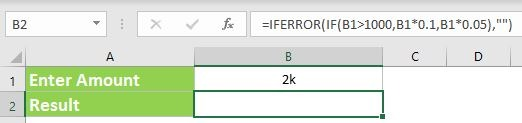Empty Argument:The following formula will result an Empty Cell if the the expression evaluates an Error. Here, we are not providing the second parameter of the IFERROR Function. We are just closing the function after the comma.

=IFERROR(IF(B1>1000,B1*0.1,B1*0.05),)Difference between Providing the Second Argument and Leaving Empty: Function creates a Blank String Character(“”), overwrites the default cell format when you pass the second character. If you leave empty, this will creates cell with default format (as if you entered nothing in the cell).

### IFERROR VLOOKUP

IFERROR is used with the combination of VLOOKUP function. VLOOKUP  function returns an error value (#NA) if it is not found the lookup value in the lookup table array range. We can suppress these errors using IFERROR function with VLOOKUP. Otherwise, we can perform another operation if Vlookup returns an Error.

Let us see an example to understand how to use IFERROR and VLOOKUP together. VLOOKUP tries to find the lookup value (D2) in the lookup table array (A2:B11) and returns the corresponding  value in column 2. If VLOOKUP not find the value in the given range, IFERROR Returns a string (“Not found”)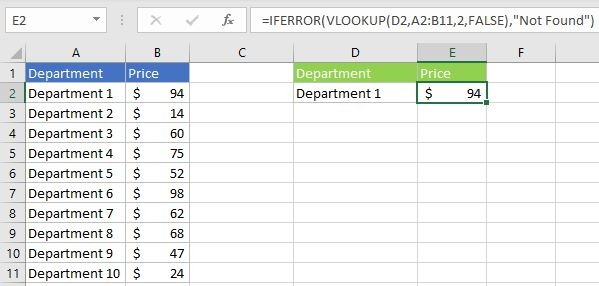### IFERROR INDEX MATCH

We use INDEX and MATCH Functions to create Advanced VLOOKUP Formula. We can use IFERROR with the combination of INDEX and MATCH functions (as used in the VLOOKUP function). INDEX and MATH Lookup functions returns an error value (#NA) if the lookup value is not found the lookup range. We can use IFERROR function to produce the alternative string.

Here is a simple example to show you how to use IFERROR function along with INDEX and MATCH reference functions.  MATCH function returns the match position of the lookup value (D4) in the lookup range (\$A\$2:\$A\$11). And the INDEX function returns the value from the reference (\$B\$2:\$B\$11) from the row number returned by MATCH function. Match returns #NA error if the given lookup value is not found in the lookup range. We can use IFERROR to Returns a new string (“Not found”)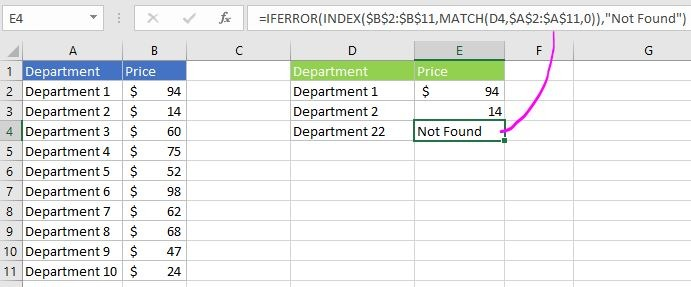### IF And IFERROR Combined

We can Combine IF and IFERROR Functions in Excel to create issue free Formulas. We can check some expression using IF Function. And trap the result using IFERROR function.

=IFERROR(IF(Sum(A1:A5)>100,”True”,”False”),”Not Valid Data in Range A1:A5″)

This formula will checks an expression using IF and returns the defined value. If it returns an error, error message will be displayed in the Cell.

### IF IFERROR and VLOOKUP

We can also Combine IF, IFERROR Functions with VLOOKUP in Excel to create advanced Formulas. VLOOKUP fetch a value from table range. And the IFERROR Evaluates the VLOOKUP output. Finally, IF functions makes the decision to display the value based on the result.

Here is real-time example to understand how to use IFERROR and VLOOKUP together in the same formula.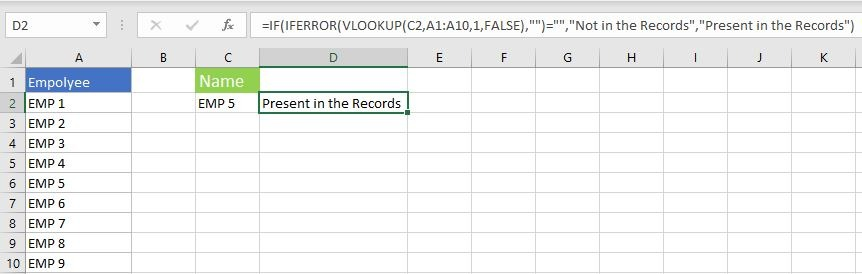=IF(IFERROR(VLOOKUP(C2,A1:A10,1,FALSE),””)=””,”Not in the Records”,”Present in the Records”)
• In this formula, VLOOKUP will check for an EMP Record
• IFERROR returns blank if no record is found
• IF statement will display the message based on the result

### Nested IFERROR

Nested IFERROR formula is helpful to fetch the values from multiple references. Following is the example of Nested IFERROR with VLOOKUP function to check for a value in two different ranges and return the corresponding column value. If the lookup values is not found in both the ranges, return custom value or a text.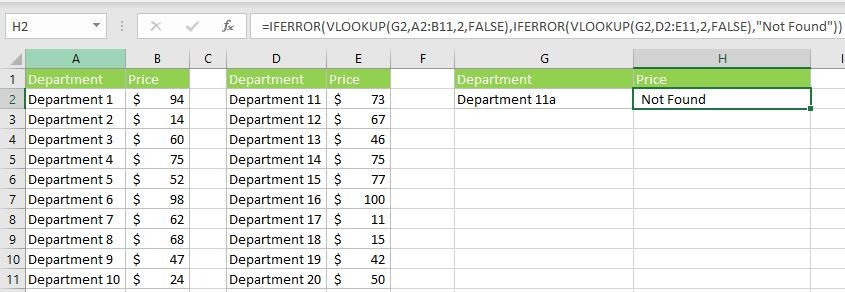• VLOOKUP(G2,A2:B11,2,FALSE) : checks for a value G2 in the first range A2:B11
• =IFERROR(VLOOKUP(G2,A2:B11,2,FALSE), : This will checks the return value and out put the corresponding value. If it is not found in the given range (A2:B11). IFERROR process the second part of the formula (i.e; IFERROR(VLOOKUP(G2,D2:E11,2,FALSE),”Not Found”))
• VLOOKUP(G2,D2:E11,2,FALSE):checks for a value G2 in the first range D2:E11
• IFERROR(VLOOKUP(G2,D2:E11,2,FALSE),”Not Found”) : This will checks the return value of 2nd VLOOKUP and out put the corresponding value. If it is not found in the given range (D2:E11). IFERROR process the second argument of the function and returns the string “Not Found”

### Nested IFERROR and IF

We can add the IF Function with Nested IFERROR function to return the value based on the result. We can use the above formula and display the value in the cell using IF Function.

Here, we have added Function to display a message as “Need to Add”,”Exist in the Table” based on the Nested IfError and If function.

#### Excel IFERROR Else

We can make use of this formula to check if an expression evaluates an Error then display some value. Else display another Value.

### Comparing IFERROR with Other Function

We have many functions to handle the Errors in Excel. Let us see the different Error trapping Functions in Excel and how they are different from IFERROR function.

#### ISERROR vs IFERROR

ISERROR Function is useful to check if an expression evaluates to an error or not. IFERROR Helps to replace that error with some value.

#### IF ISERROR vs IFERROR

If ISERROR combination is useful when you wants to check an expression and execute Expression One when it is True and Expression Two when it is False.

#### IFNA vs IFERROR

IFNA Function is used when you wants to replace only if an expression returns #NA Error. Where as IFERROR is helps to tackle with any type of errors listed above.

#### ISNA vs IFERROR

ISNA Function is used to check if an expression is Returning a #NA type Error. IFERROR is used to replace an error if the expression evaluates to any error listed above.

#### IF and IFERROR

IF Function is used to check a condition and executes the first expression when it is True and the second expression when it is False. But IFERROR is used when you want to replace an error only if the expression evaluates to any error listed above.

#### IFERROR vs IFNA for VLOOKUP

VlookUp returns #NA type error if the lookup value is not found in the table array. You can use IFNA function to deal with this situation.  But, in some cases the VlookUp return other type of Errors like  #Name (based on the input expressions). You can use IfError to deal with any type of Errors as mention above.

### How Does IFERROR Work

IFERROR Works as error handling formula in Excel. Following is the process flow chart of IFERROR Function with clear instructions.• It required two arguments to process the function
• It will process the first argument and validate the evaluated value
• If the first argument returns the value which is not an error (#VALUE!,#N/A, #REF!, #DIV/0!, #NUM! #NAME? and #NULL!.), then it returns the result output of the first argument
• If the first argument returns an error, then it will process the second argument of the IFERROR function and returns the output

Here are the simple examples to understand how does IFERROR function Works. The first example returns the actual value, and the second example returns the IFERROR function value.

Example 1: We have provided the First argument as 25+75, tried to add number and number. It returns the values as 100. So, the IFERROR function Returns the first argument.

Formula

=IFERROR(25+75 ,”You can not add a number and Text”)

Output:100

Example 2: We have provided the First argument as 25+”Some Text”, tried to add number and string. It returns an Error. So, the IFERROR function Returns the second argument.

Formula

=IFERROR(25+”Some Text” ,”You can not add a number and Text”)

Output:”You can not add a number and Text”

### IFERROR Examples

#### 1. Display Message to User

Here is a simple example to explain the IFERROR Function. The following Formula check the first expression and returns the given string as it evaluates an Error.

Formula:

We can see the first argument of the formula (5/0) evaluates #DIV/0! Error. And IFERROR function trap this error and returns the second argument.

#### 2. IFERROR Then Return 0

Let us say we are performing a calculation based on the result. If there is any Error in the Formula, we want the Excel to return Zero. In this case we can use iferror and simply return 0 if the formula evaluates to an Error.

Formula:

=IFERROR(A1/B1,0)

Returns: 0 (if the formula evaluates to an error)

#### 3. IFERROR, Make the Cell Blank

Excel sheet do not look good if there are many Error Cells . We can use the IFERROR formula to return a blank if the formula returns errors.

Formula:

=IFERROR(A1/B1,””)

Returns: “” (if the formula evaluates to an error)

#### 4. Check if a value found in List of values

IFEEROR helps to determine if an item found in the list of items, range or Array. The following Example checks if value find in a range of values.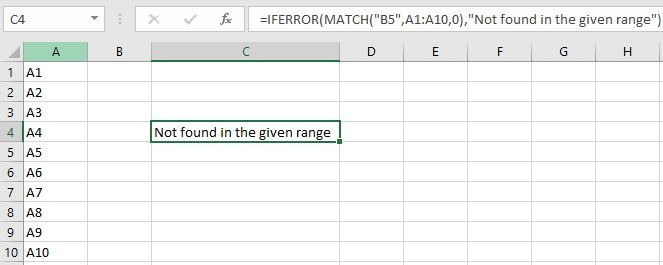#### 5. If Error then Evaluate another Formula

The following formula checks for a value in Range A1:A10. If it is not found in the A1:B10, IFERROR helps to check in another Range(D1:E10). Here is a simple example on IFERROR to return a value from one of the Ranges.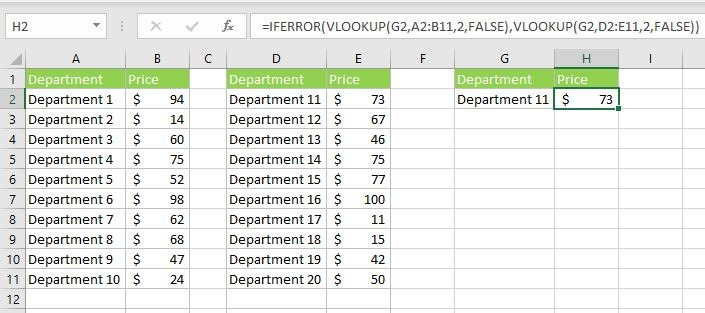### IFERROR Return Values

Excel IFERROR can be used to return values based on our requirement. We can return a string, a number, or evaluate a formula. Let us see the most widely returning Values of IFERROR Function.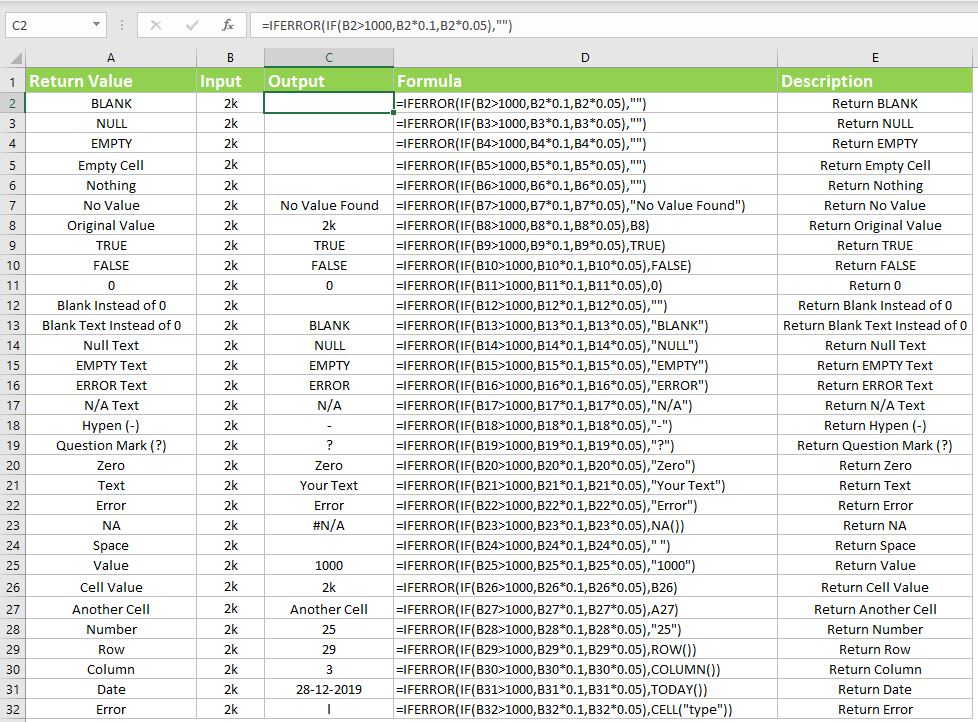Return Value Formula Description
BLANK =IFERROR(IF(B2>1000,B2*0.1,B2*0.05),””) Return BLANK

IfError Then BLANK

NULL =IFERROR(IF(B3>1000,B3*0.1,B3*0.05),””) Return NULL

IfError Then NULL

EMPTY =IFERROR(IF(B4>1000,B4*0.1,B4*0.05),””) Return EMPTY

IfError Then EMPTY

Empty Cell =IFERROR(IF(B5>1000,B5*0.1,B5*0.05),””) Return Empty Cell

IfError Then Empty Cell

Nothing =IFERROR(IF(B6>1000,B6*0.1,B6*0.05),””) Return Nothing

IfError Then Nothing

No Value =IFERROR(IF(B7>1000,B7*0.1,B7*0.05),”No Value Found”) Return No Value

IfError Then No Value

Original Value =IFERROR(IF(B8>1000,B8*0.1,B8*0.05),B8) Return Original Value

IfError Then Original Value

TRUE =IFERROR(IF(B9>1000,B9*0.1,B9*0.05),TRUE) Return TRUE

IfError Then TRUE

FALSE =IFERROR(IF(B10>1000,B10*0.1,B10*0.05),FALSE) Return FALSE

IfError Then FALSE

0 =IFERROR(IF(B11>1000,B11*0.1,B11*0.05),0) Return 0

IfError Then 0

IfError Then Blank Instead of 0

Blank Text Instead of 0 =IFERROR(IF(B13>1000,B13*0.1,B13*0.05),”BLANK”) Return Blank Text Instead of 0

IfError Then Blank Text Instead of 0

Null Text =IFERROR(IF(B14>1000,B14*0.1,B14*0.05),”NULL”) Return Null Text

IfError Then Null Text

EMPTY Text =IFERROR(IF(B15>1000,B15*0.1,B15*0.05),”EMPTY”) Return EMPTY Text

IfError Then EMPTY Text

ERROR Text =IFERROR(IF(B16>1000,B16*0.1,B16*0.05),”ERROR”) Return ERROR Text

IfError Then ERROR Text

N/A Text =IFERROR(IF(B17>1000,B17*0.1,B17*0.05),”N/A”) Return N/A Text

IfError Then N/A Text

Hyphen (-) =IFERROR(IF(B18>1000,B18*0.1,B18*0.05),”-“) Return Hyphen (-)

IfError Then Hyphen (-)

Question Mark (?) =IFERROR(IF(B19>1000,B19*0.1,B19*0.05),”?”) Return Question Mark (?)

IfError Then Question Mark (?)

Zero =IFERROR(IF(B20>1000,B20*0.1,B20*0.05),”Zero”) Return Zero

IfError Then Zero

IfError Then Text

Error =IFERROR(IF(B22>1000,B22*0.1,B22*0.05),”Error”) Return Error

IfError Then Error

NA =IFERROR(IF(B23>1000,B23*0.1,B23*0.05),NA()) Return NA

IfError Then NA

Space =IFERROR(IF(B24>1000,B24*0.1,B24*0.05),” “) Return Space

IfError Then Space

Value =IFERROR(IF(B25>1000,B25*0.1,B25*0.05),”1000″) Return Value

IfError Then Value

Cell Value =IFERROR(IF(B26>1000,B26*0.1,B26*0.05),B26) Return Cell Value

IfError Then Cell Value

Another Cell =IFERROR(IF(B27>1000,B27*0.1,B27*0.05),A27) Return Another Cell

IfError Then Another Cell

Number =IFERROR(IF(B28>1000,B28*0.1,B28*0.05),”25″) Return Number

IfError Then Number

Row =IFERROR(IF(B29>1000,B29*0.1,B29*0.05),ROW()) Return Row

IfError Then Row

Column =IFERROR(IF(B30>1000,B30*0.1,B30*0.05),COLUMN()) Return Column

IfError Then Column

Date =IFERROR(IF(B31>1000,B31*0.1,B31*0.05),TODAY()) Return Date

IfError Then Date

Error =IFERROR(IF(B32>1000,B32*0.1,B32*0.05),CELL(“type”)) Return Error

IfError Then Error

#### Return 0:

We can use IFERROR to return 0 if the formula evaluates an Error. This is very helpful while processing the numerical data. If you have the formulas witch are resulting an Error while calculation, it will effect the all other calculations. If you return 0 using IFERROR, you can avoid those issues with your spreadsheet.

Formula:

#### Return Blank:

If you are dealing with string data, you can return blank instead of 0. It makes your sheet more cleaner and issue free. Following is the formula to Return Blank if there are any errors in Formula.

Formula:

#### Return Null:

You can make use of IFERROR and return Null string to avoid conflicts with other formula. Here is

### Best Practices

Please remember the following best practices to be followed while using IFERROR in Excel Formula.

• IFERROR is very important and useful formula to create an issue free spreadsheet worksheet. Use this formula when there is a chance of evaluation error.
• Try to use smaller portion of the formula, instead of the entire formula.
• Do not leave the arguments empty. IFERROR treats the empty arguments as an Empty String (“”).
• Try to Avoid using IF  + ISERROR , instead use IFERROR Function
• Use ISNA function to check if the expression evaluates only #NA Error, otherwise use IFERROR to catch all Errors
• ISERR function to check if the expression evaluates errors except #NA Error